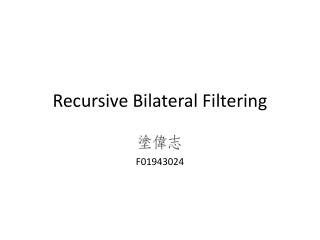DownloadDownload PresentationRecursive Bilateral Filtering

# Recursive Bilateral Filtering

Download Presentation## Recursive Bilateral Filtering

- - - - - - - - - - - - - - - - - - - - - - - - - - - E N D - - - - - - - - - - - - - - - - - - - - - - - - - - -
##### Presentation Transcript

1. Recursive Bilateral Filtering F01943024

2. Reference • Yang, Qingxiong. "Recursive bilateral filtering." ECCV 2012. • Deriche, Rachid. "Recursively implementating the Gaussian and its derivatives." ICIP 1993.

3. Outline • Recap of Bilateral Filtering • Why Recursive Filtering? • Recursive Bilateral Filtering • Complexity Analysis • Applications • Conclusion

4. Recap of Bilateral Filtering • Naïve image smoothing: Gaussian filtering • Not edge-preserving • Bilateral filtering

5. Recap of Bilateral Filtering • The bilateral filter is a robust edge-preserving filter introduced by Tomasi and Manduchi in 1998.

6. Recap of Bilateral Filtering • Being non-linear, the brute force implementations of the bilateral filter are slow when kernel is large. • Pham and Vliet implemented the BF as a separable operation.The cost is still high for large kernels. (In ICME 2005) • By constraining the spatial filter kernel to box filter, Weiss showed that the result depends only on the histogram of the neighborhood. However, this method works efficiently only on grascale image. (In Siggraph 2006) • Paris and Durand presented a volumetric data structure called bilateral grid. The BF corresponds to convolving a grid with a 3D/5D Gaussian. However, the memory cost maybe unacceptable when filter kernel is small. (In Siggraph 2007)

7. Recap of Bilateral Filtering • Porikli is the first to remove the dependency of the filter(kernel) size by integral histogram. He also proposed a Taylor series based solution to remove the box filter constraint. (In CVPR 2008) • Yang showed that Durand’s method can be implemented using recursive Gaussian filter so that its complexity will be independent of the filter size. (In CVPR 2009) • The state-of-the-art implementation is proposed by Adams. • Gaussian KD-trees, complexity O(Nlog(N)D), memory cost O(ND) • Permutohedral lattice, complexity O(ND2), memory cost much higher • This paper: implementing bilateral filter in a recursive fasion with complexity O(ND), memory cost O(ND)

8. Why Recursive Filtering? • FIR filter • IIR filter

9. Why Recursive Filtering? • 1st-order recursive filtering • 2nd-order recursive filtering Ex: [Deriche 1992] demonstrated that Gaussian filter can be computed using 2nd-order recursive filtering.

10. Why Recursive Filtering? • Deriche, R. Recursively implementing the Gaussian and its derivatives. In ICIP. 1992 causal anticausal

11. Why Recursive Filtering? • So why? • Filter size dependency • Recursive implementation is linear in the number of pixels.

12. Recursive Bilateral Filtering • Bilateral filtering • Modified range kernel • The proposed method measures the range distance by accumulating the color difference between every two neighboring pixels on the path between k and i.

13. Recursive Bilateral Filtering Claim: For any bilateral filter containing the new range filter kernel and any spatial filter kernel that can be recursively implemented, an exact recursive implementation can be obtained by simply altering the coefficients of the recursive system defined by the spatial filter kernel at each pixel location. • Recursive implementation of the spatial filter • Recursive bilateral filter Con be proved by math induction: http://www.cs.cityu.edu.hk/~qiyang/publications/eccv-12/

14. Complexity Analysis • Recursive implementation of the spatial filter • Recursive bilateral filter • New range kernel can be computed recursively. 2n multiplication operations and 2n-1 addition/subtraction operations Only 3n-2 additional multiplication operations

15. Applications • Non-Photorealistic Rendering

16. Applications • Tone Mapping

17. Applications • Stereo Matching (average 10x faster)

18. Conclusion • A recursive implementation of the bilateral filter is proposed in this paper. With • a new range filter kernel • any spatial filter kernel that can be recursively implemented. • It’s the first bilateral whose computational and memory complexity are linear in both input size and dimensionality.